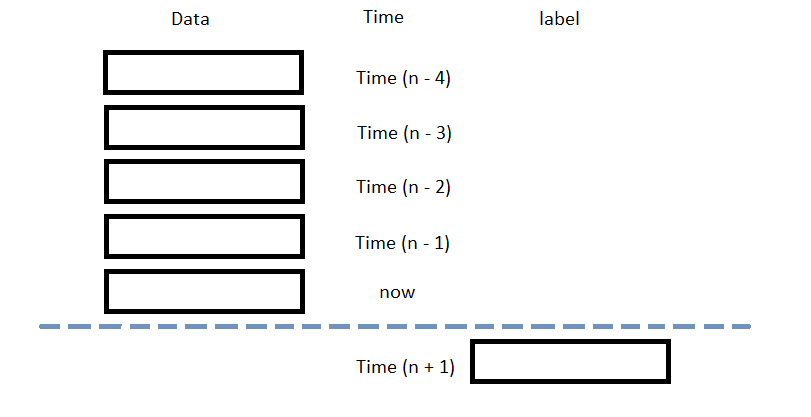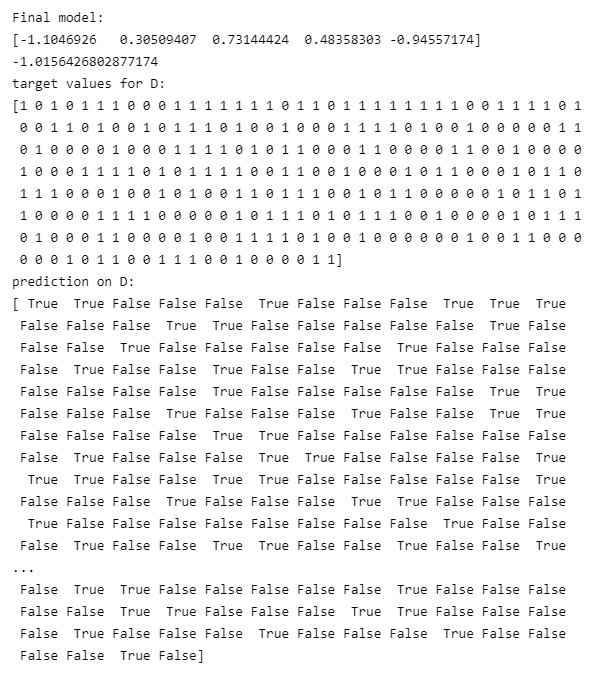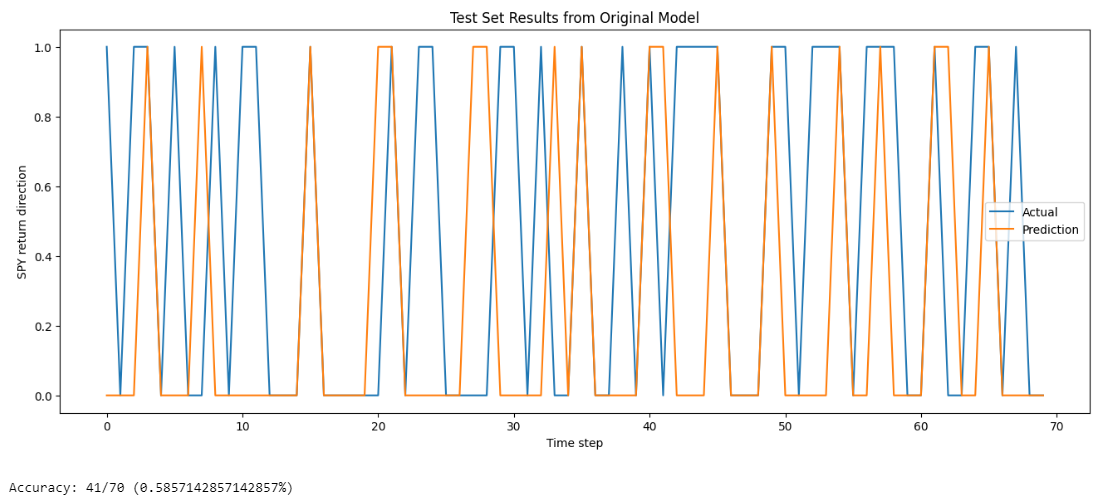# Machine Learning

## Aesera

### Introduction

This page explains how to build, train, test, and store Aesera models.

### Import Libraries

Import the aesera, and sklearn libraries.

import aesara
import aesara.tensor as at
from sklearn.model_selection import train_test_split
from sklearn.preprocessing import MinMaxScaler
import joblib

You need the joblib library to store models.

### Get Historical Data

Get some historical market data to train and test the model. For example, to get data for the SPY ETF during 2020 and 2021, run:

qb = QuantBook()
history = qb.History(symbol, datetime(2020, 1, 1), datetime(2022, 1, 1)).loc[symbol]

### Prepare Data

You need some historical data to prepare the data for the model. If you have historical data, manipulate it to train and test the model. In this example, use the following features and labels:

Data CategoryDescription
FeaturesNormalized close price of the SPY over the last 5 days
LabelsReturn direction of the SPY over the next day

The following image shows the time difference between the features and labels:Follow these steps to prepare the data:

1. Obtain the close price and return direction series.
2. close = history['close']
returns = data['close'].pct_change().shift(-1)[lookback*2-1:-1].reset_index(drop=True)
labels = pd.Series([1 if y > 0 else 0 for y in returns])   # binary class
3. Loop through the close Series and collect the features.
4. lookback = 5
lookback_series = []
for i in range(1, lookback + 1):
df = data['close'].shift(i)[lookback:-1]
df.name = f"close-{i}"
lookback_series.append(df)
X = pd.concat(lookback_series, axis=1)
# Normalize using the 5 day interval
X = MinMaxScaler().fit_transform(X.T).T[4:]
5. Convert the lists of features and labels into numpy arrays.
6. X = np.array(features)
y = np.array(labels)
7. Split the data into training and testing periods.
8. X_train, X_test, y_train, y_test = train_test_split(X, y, test_size=0.2)

### Train Models

You need to prepare the historical data for training before you train the model. If you have prepared the data, build and train the model. In this example, build a Logistic Regression model with log loss cross entropy and square error as cost function. Follow these steps to create the model:

1. Generate a dataset.
2. # D = (input_values, target_class)
D = (np.array(X_train), np.array(y_train))
3. Initialize variables.
4. # Declare Aesara symbolic variables
x = at.dmatrix("x")
y = at.dvector("y")

# initialize the weight vector w randomly using share so model coefficients keep their values
rng = np.random.default_rng(100)
w = aesara.shared(rng.standard_normal(X.shape), name="w")

# initialize the bias term
b = aesara.shared(0., name="b")
5. Construct the model graph.
6. # Construct Aesara expression graph
p_1 = 1 / (1 + at.exp(-at.dot(x, w) - b))       # Logistic transformation
prediction = p_1 > 0.5                          # The prediction thresholded
xent = y * at.log(p_1) - (1 - y) * at.log(1 - p_1)  # Cross-entropy log-loss function
cost = xent.mean() + 0.01 * (w ** 2).sum()      # The cost to minimize (MSE)
gw, gb = at.grad(cost, [w, b])                  # Compute the gradient of the cost
7. Compile the model.
8. train = aesara.function(
inputs=[x, y],
outputs=[prediction, xent],
updates=((w, w - 0.1 * gw), (b, b - 0.1 * gb)))
predict = aesara.function(inputs=[x], outputs=prediction)
9. Train the model with training dataset.
10. pred, err = train(D, D)

# We can also inspect the final outcome
print("Final model:")
print(w.get_value())
print(b.get_value())
print("target values for D:")
print(D)
print("prediction on D:")
print(predict(D))    # whether > 0.5 or not### Test Models

You need to build and train the model before you test its performance. If you have trained the model, test it on the out-of-sample data. Follow these steps to test the model:

1. Call the predict method with the features of the testing period.
2. y_hat = predict(np.array(X_test))
3. Plot the actual and predicted labels of the testing period.
4. df = pd.DataFrame({'y': y_test, 'y_hat': y_hat}).astype(int)
df.plot(title='Model Performance: predicted vs actual return direction in closing price', figsize=(12, 5))
5. Calculate the prediction accuracy.
6. correct = sum([1 if x==y else 0 for x, y in zip(y_test, y_hat)])
print(f"Accuracy: {correct}/{y_test.shape} ({correct/y_test.shape}%)")### Store Models

You can save and load aesera models using the Object Store.

#### Save Models

Follow these steps to save models in the Object Store:

1. Set the key name of the model to be stored in the Object Store.
2. model_key = "model"
3. Call the GetFilePath method with the key.
4. file_name = qb.ObjectStore.GetFilePath(model_key)

This method returns the file path where the model will be stored.

5. Call the dump method with the model and file path.
6. joblib.dump(predict, file_name)

If you dump the model using the joblib module before you save the model, you don't need to retrain the model.

You must save a model into the Object Store before you can load it from the Object Store. If you saved a model, follow these steps to load it:

1. Call the ContainsKey method with the model key.
2. qb.ObjectStore.ContainsKey(model_key)

This method returns a boolean that represents if the model_key is in the Object Store. If the Object Store does not contain the model_key, save the model using the model_key before you proceed.

3. Call GetFilePath with the key.
4. file_name = qb.ObjectStore.GetFilePath(model_key)

This method returns the path where the model is stored.

5. Call load with the file path.
6. loaded_model = joblib.load(file_name)

This method returns the saved model.

You can also see our Videos. You can also get in touch with us via Discord.